# Force on current carrying conductorForce on a current carrying conductor

When a charged particle moves in a magnetic field it experiences force. Similarly, when an electron moves in a magnetic field it experiences force. We all know that electric current in a conductor is due to the drifting of the free electrons in a definite direction. So, when a current carrying conductor is placed in a magnetic field, the drifting free electrons experience force. As, these electrons are within the conductor we can say that the magnetic field applies force on the conductor. So, let’s discuss about the force acting on the conductor.

Expression of forceLet’s suppose a conductor of length  ­­and crosssectional area A placed at an angle θ in a uniform magnetic field B as shown in the figure. Let the current flowing through the conductor be I, vbe the drift velocity of the free electrons flowing through the conductor, n be the electron density of the conductor and e be the charge of an electron.

We know force on each electron is given by :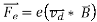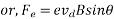Altogether, there are nAfree electrons in the lengthof the conductor where Ais volume of the conductor. So magnitude of total force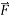acting along­ the conductor is given by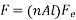­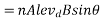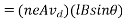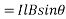Where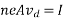is the current flowing through the conductor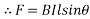In vector form, the Lorentz force can be written as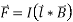The vectoris taken in the direction of the current. The direction of this force is perpendicular to the plane containingand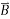. It can be found by using Fleming’s left hand rule or right hand palm rule.

Reference take from Principle of Physics – Ayam Publication.